# Buy and Sell Stock – II | (DP -36)

Problem Statement:

Buy and Sell Stock – II

We are given an array Arr[] of length n. It represents the price of a stock on ‘n’ days. The following guidelines need to be followed:

1. We can buy and sell the stock any number of times.
2. In order to sell the stock, we need to first buy it on the same or any previous day.
3. We can’t buy a stock again after buying it once. In other words, we first buy a stock and then sell it. After selling we can buy and sell again. But we can’t sell before buying and can’t buy before selling any previously bought stock.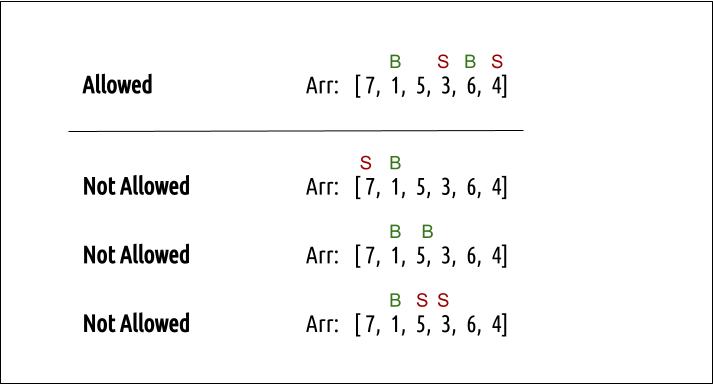Example: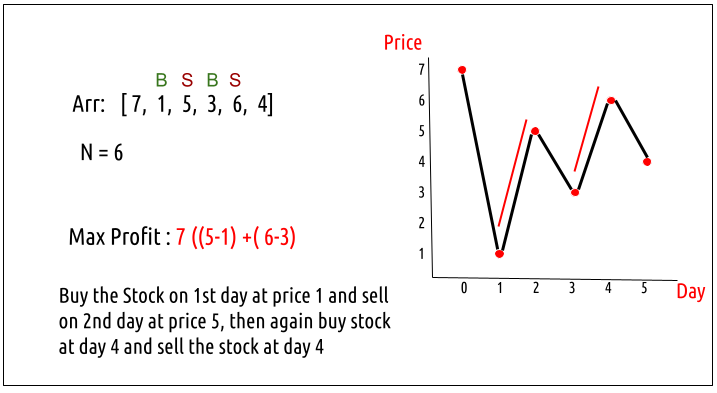Disclaimer: Don’t jump directly to the solution, try it out yourself first.

### Solution :

Intuition:

Every day, we will have two choices, either to do nothing and move to the next day or to buy/sell (based on the last transaction) and find out the profit. Therefore we need to generate all the choices in order to compare the profit. As we need to try out all the possible choices, we will use recursion.

Steps to form the recursive solution:

We will first form the recursive solution by the three points mentioned in the Dynamic Programming Introduction

Step 1: Express the problem in terms of indexes.

We need to think in the terms of the number of days, therefore one variable will be the array index( say ind). Next, we need to respect the condition that we can’t buy a stock again, that is we need to first sell a stock, and then we can buy that again. Therefore we need a second variable ‘buy’ which tells us on a particular day whether we can buy or sell the stock. We can generalize the function as :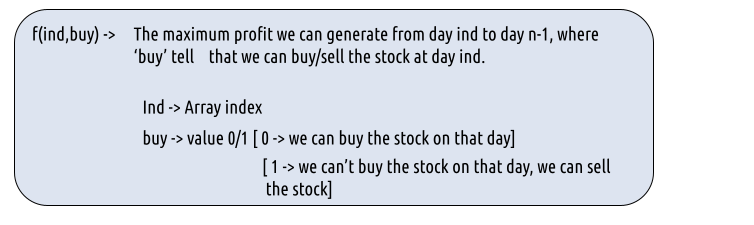Step 2: Try out all possible choices at a given index.

Every day, we have two choices:

• To do nothing and move on to the next day.

We need to generate all the choices. We will use the pick/non-pick technique as discussed in this video “Recursion on Subsequences”.

If we can buy the stock on a particular day, we have two options:

• Option 1: To do no transaction and move to the next day. In this case, the net profit earned will be 0 from the current transaction, and to calculate the maximum profit starting from the next day, we will recursively call f(ind+1,0). As we have not bought the stock, the ‘buy’ variable value will still remain 0, indicating that we can buy the stock the next day.
• Option 2: The other option is to buy the stock on the current day. In this case, the net profit earned from the current transaction will be -Arr[i]. As we are buying the stock, we are giving money out of our pocket, therefore the profit we earn is negative. To calculate the maximum profit starting from the next day, we will recursively call f(ind+1,1). As we have bought the stock, the ‘buy’ variable value will change to 1, indicating that we can’t buy and only sell the stock the next day.

Case 2: When buy == 1, we can sell the stock.

If we can buy the stock on a particular day, we have two options:

• Option 1: To do no transaction and move to the next day. In this case, the net profit earned will be 0 from the current transaction, and to calculate the maximum profit starting from the next day, we will recursively call f(ind+1,1). As we have not bought the stock, the ‘buy’ variable value will still remain at 1, indicating that we can’t buy and only sell the stock the next day.
• Option 2: The other option is to sell the stock on the current day. In this case, the net profit earned from the current transaction will be +Arr[i]. As we are selling the stock, we are putting the money into our pocket, therefore the profit we earn is positive. To calculate the maximum profit starting from the next day, we will recursively call f(ind+1,0). As we have sold the stock, the ‘buy’ variable value will change to 0, indicating that we can buy the stock the next day.

The figure below gives us the summary: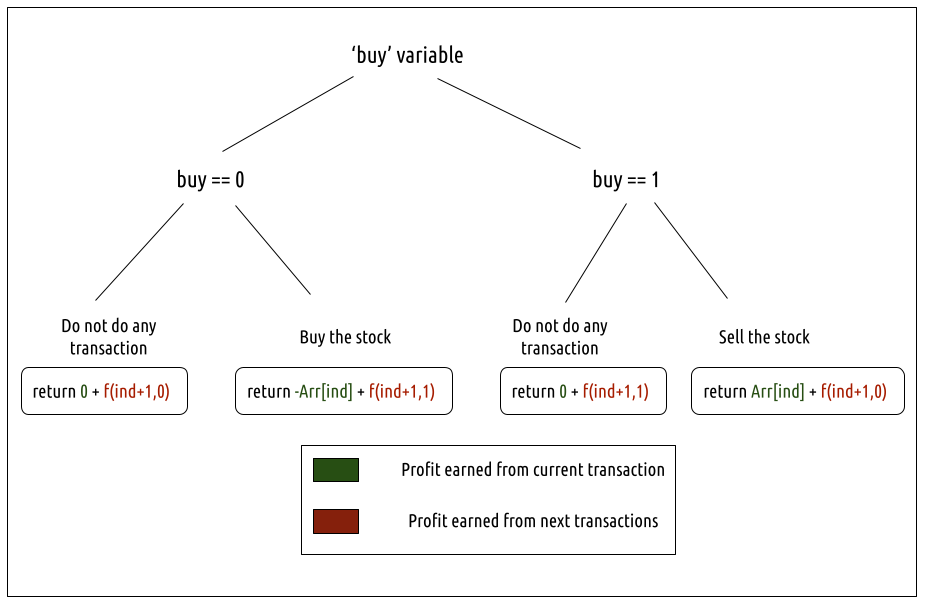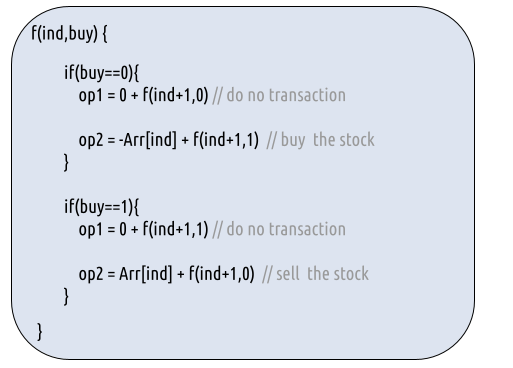Step 3:  Return the maximum

As we are looking to maximize the profit earned, we will return the maximum value in both cases.

The final pseudocode after steps 1, 2, and 3: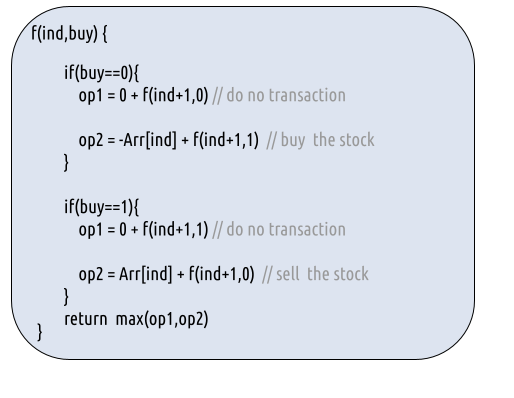Base Cases:

• If ind==n, it means we have finished trading on all days, and there is no more money that we can get, therefore we simply return 0.

Steps to memoize a recursive solution:

If we draw the recursion tree, we will see that there are overlapping subproblems. In order to convert a recursive solution the following steps will be taken:

1. Create a dp array of size [n]. The size of the input array is ‘n’, so the index will always lie between ‘0’ and ‘n-1’. The ‘buy’  variable can take only two values: 0 and 1. Therefore we take the dp array as dp[n]
2. We initialize the dp array to -1.
3. Whenever we want to find the answer to particular parameters (say f(ind, buy)), we first check whether the answer is already calculated using the dp array(i.e dp[ind][buy]!= -1 ). If yes, simply return the value from the dp array.
4. If not, then we are finding the answer for the given value for the first time, we will use the recursive relation as usual but before returning from the function, we will set dp[ind][buy] to the solution we get.

Code:

## C++ Code

``````#include <bits/stdc++.h>

using namespace std;

long getAns(long *Arr, int ind, int buy, int n, vector<vector<long>> &dp ){

if(ind==n) return 0; //base case

long profit;

profit = max(0+getAns(Arr,ind+1,0,n,dp), -Arr[ind] + getAns(Arr,ind+1,1,n,dp));
}

if(buy==1){// We can sell the stock
profit = max(0+getAns(Arr,ind+1,1,n,dp), Arr[ind] + getAns(Arr,ind+1,0,n,dp));
}

}

long getMaximumProfit(long *Arr, int n)
{

vector<vector<long>> dp(n,vector<long>(2,-1));

if(n==0) return 0;
long ans = getAns(Arr,0,0,n,dp);
return ans;
}

int main() {

int n =6;
long Arr[n] = {7,1,5,3,6,4};

cout<<"The maximum profit that can be generated is "<<getMaximumProfit(Arr, n);
}
``````

Output:

The maximum profit that can be generated is 7

Time Complexity: O(N*2)

Reason: There are N*2 states therefore at max ‘N*2’ new problems will be solved and we are running a for loop for ‘N’ times to calculate the total sum

Space Complexity: O(N*2) + O(N)

Reason: We are using a recursion stack space(O(N)) and a 2D array ( O(N*2)).

Steps to convert Recursive Solution to Tabulation one.

To convert the memoization approach to a tabulation one, create a dp array with the same size as done in memoization. We can set its type as bool and initialize it as false.

• First, we declare the dp array of size [n+1] as zero.
• Next, we set the base condition, we set dp[n] = dp[n] = 0(the case when we had exhausted the number of days of the stock market).
• Next, we set two nested for loops, the outer loop (for variable ind) moves from  n-1 to 0 and the inner loop (for variable buy) moves from 0 to 1.
• In every iteration, we calculate the value according to the memoization logic.
• At last, we will print dp as our answer.

Code:

## C++ Code

``````#include <bits/stdc++.h>

using namespace std;

long getMaximumProfit(long *Arr, int n)
{

vector<vector<long>> dp(n+1,vector<long>(2,-1));

//base condition
dp[n] = dp[n] = 0;

long profit;

for(int ind= n-1; ind>=0; ind--){
profit = max(0+dp[ind+1], -Arr[ind] + dp[ind+1]);
}

if(buy==1){// We can sell the stock
profit = max(0+dp[ind+1], Arr[ind] + dp[ind+1]);
}

}
}
return dp;
}

int main() {

int n =6;
long Arr[n] = {7,1,5,3,6,4};

cout<<"The maximum profit that can be generated is "<<getMaximumProfit(Arr, n);
}
``````

Output:

The maximum profit that can be generated is 7

Time Complexity: O(N*2)

Reason: There are two nested loops that account for O(N*2) complexity.

Space Complexity: O(N*2)

Reason: We are using an external array of size ‘N*2’. Stack Space is eliminated.

## Java Code

``````import java.util.*;

class TUF{
static long getMaximumProfit(long Arr[], int n)
{

long dp[][]=new long[n+1];
for(long row[]: dp)
Arrays.fill(row,-1);

//base condition
dp[n] = dp[n] = 0;

long profit=0;

for(int ind= n-1; ind>=0; ind--){
profit = Math.max(0+dp[ind+1], -Arr[ind] + dp[ind+1]);
}

if(buy==1){// We can sell the stock
profit = Math.max(0+dp[ind+1], Arr[ind] + dp[ind+1]);
}

}
}
return dp;
}

public static void main(String args[]) {

int n =6;
long Arr[] = {7,1,5,3,6,4};

System.out.println("The maximum profit that can be generated is "+getMaximumProfit(Arr, n));
}
}``````

Output:

The maximum profit that can be generated is 7

Time Complexity: O(N*2)

Reason: There are two nested loops that account for O(N*2) complexity.

Space Complexity: O(N*2)

Reason: We are using an external array of size ‘N*2’. Stack Space is eliminated.

Part 3: Space Optimization

If we closely look the relation,

We see that to calculate a value of a cell of the dp array, we need only the next column values(say ahead). So, we don’t need to store an entire 2-D array. Hence we can space optimize it.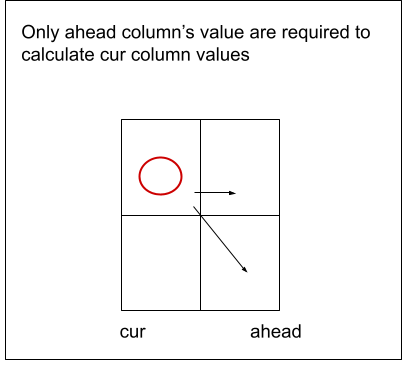• We set the ahead column as 0 (base condition)
• Then we set the nested loops to calculate the cur column values.
• We replace dp[ind] with cur and dp[ind+1] with ahead in our memoization code.
• After the inner loop execution, we set ahead as cur for the next outer loop iteration.
• At last, we return cur as our answer.

Code:

## C++ Code

``````#include <bits/stdc++.h>

using namespace std;

long getMaximumProfit(long *Arr, int n)
{

vector<long> cur(2,0);

//base condition

long profit;

for(int ind= n-1; ind>=0; ind--){
}

if(buy==1){// We can sell the stock
}
}

}
return cur;
}

int main() {

int n =6;
long Arr[n] = {7,1,5,3,6,4};

cout<<"The maximum profit that can be generated is "<<getMaximumProfit(Arr, n);
}
``````

Output:

The maximum profit that can be generated is 7

Time Complexity: O(N*2)

Reason: There are two nested loops that account for O(N*2) complexity

Space Complexity: O(1)

Reason: We are using an external array of size ‘2’.

## Java Code

``````import java.util.*;

class TUF{
static long getMaximumProfit(long Arr[], int n)
{

long cur[] =new long;

//base condition

long profit=0;

for(int ind= n-1; ind>=0; ind--){
}

if(buy==1){// We can sell the stock
}
}

}
return cur;
}

public static void main(String args[]) {

int n =6;
long Arr[] = {7,1,5,3,6,4};

System.out.println("The maximum profit that can be generated is "+
getMaximumProfit(Arr, n));
}
}``````

Output:

The maximum profit that can be generated is 7

Time Complexity: O(N*2)

Reason: There are two nested loops that account for O(N*2) complexity

Space Complexity: O(1)

Reason: We are using an external array of size ‘2’.